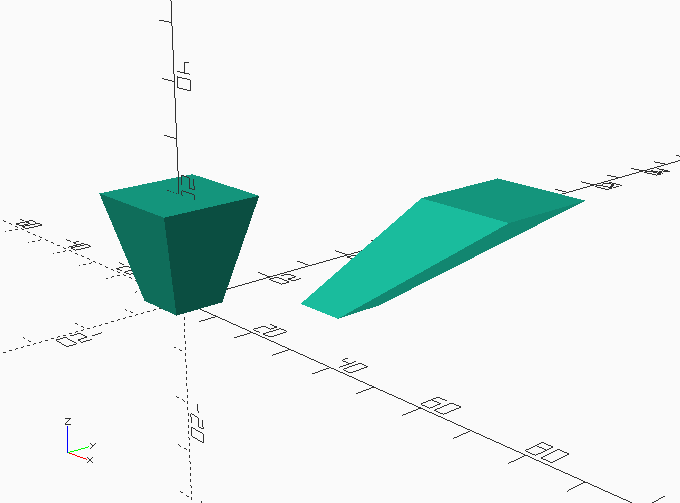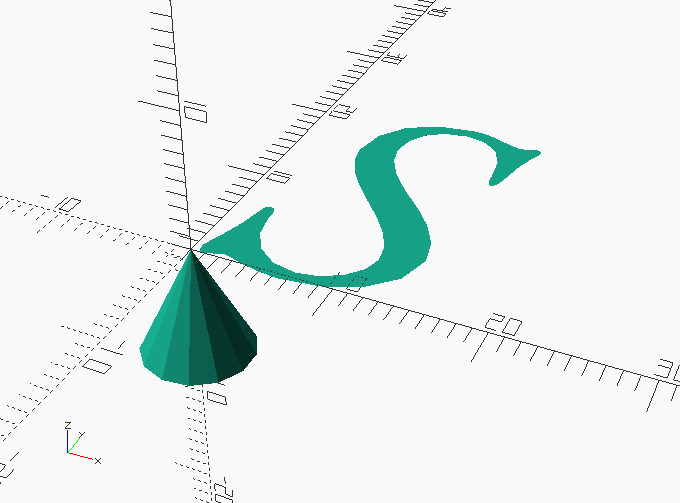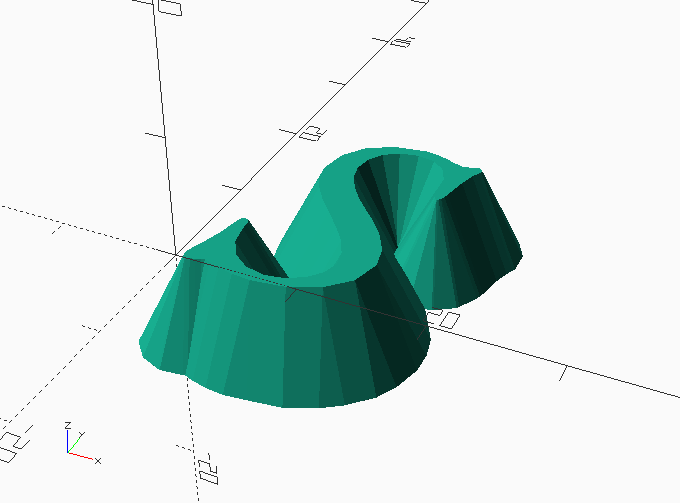Categories

# Symmetric chamfered extrusion in OpenSCAD

I like using OpenSCAD, but it has some limitations. While you can linear_extrude() 2D paths into 3D shapes, you can’t get a proper tapered/chamfered extrusion of anything but simple shapes that are symmetric about the origin:// this is symmetrical … linear_extrude(height=20, scale=2)square(10, center=true); // but shift the same square off the origin and this happens … linear_extrude(height=20, scale=2)translate([20, 20])square(10, center=true);

There are lots of partial attempts at fixing this, many of which end up with ugly results. Some of them even mess up the top surface, which is precisely what I wanted to avoid. My code uses the computationally-intensive minkowski() sum function to replace every vertex of a 2D shape with a many-sided pyramid.

Minkowski sums effectively replace every vertex with another shape, here making a rounded cube from a cube and a sphere:

One feature of OpenSCAD’s implementation of the Minkowski sum is that the operator takes into account the second shape’s position relative to the origin. So if I take the same cube and apply the minkowski() operator with the same sphere moved away from the origin, I get:

So I can approximate a tapered extrusion by turning a 2d path into a very thin 3d plate (OpenSCAD’s 2D and 3D subsystems can never meet in the same output) and using a pyramid as the second argument to the operator:// the component parts, before minkowski()// thin extrusion of 2D pathlinear_extrude(height=0.001)text(“S”, size=24, font=”EB Garamond:style=12 Italic”);// a 30 degree pyramid with its apex at the originrotate_extrude()polygon([ [0,0] , [4, -8], [0, -8] ]);

You get:minkowski() { // thin extrusion of 2D path linear_extrude(height=0.001)text(“S”, size=24, font=”EB Garamond:style=12 Italic”); // a 30 degree pyramid with its apex at the origin rotate_extrude()polygon([ [0,0] , [4, -8], [0, -8] ]); }

In reality, you’d probably use a smaller taper angle, but the example is short rather than pretty. If you’re really picky about correctness, the process leaves the thin extrusion as parallel walls at the bottom of the shape, shown grossly exaggerated here for effect:

`include <chamfer_extrude.scad>; chamfer_extrude(height=4, angle=15, \$fn=16)text("S", size=24, font="EB Garamond:style=12 Italic", \$fn=64);`